# Science, Nature & Maths Mathematics Books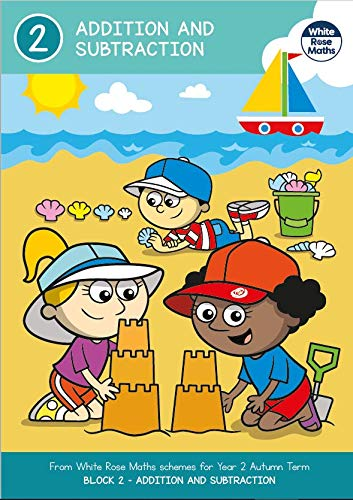## Year 2 ADDITION AND SUBTRACTION Autumn Term BLOCK 2 - White Rose Maths

From White Rose Maths schemes for Year 2 Autumn Term BLOCK 2 – ADDITION AND SUBTRACTION. The activities are designed to meet the aims of the national curriculum for mathematics: - become fluent in the fundamentals of mathematics, including through varied and frequent practice with increasingly complex problems over time, so that pupils develop conceptual understanding and the ability to recall and apply knowledge rapidly and accurately. - reason mathematically by following a line of enquiry, conjecturing relationships and generalisations, and developing an argument, justification or proof using mathematical language - can solve problems by applying their mathematics to a variety of routine and non-routine problems with increasing sophistication, including breaking down problems into a series of simpler steps and persevering in seeking solutions. Curriculum link for Year 2: - solve problems with addition and subtraction: ○ using concrete objects and pictorial representations, including those involving numbers, quantities and measures ○ applying their increasing knowledge of mental and written methods - recall and use addition and subtraction facts to 20 fluently, and derive and use related facts up to 100 - add and subtract numbers using concrete objects, pictorial representations, and mentally, including: ○ a two-digit number and ones ○ a two-digit number and tens ○ two two-digit numbers ○ adding three one-digit numbers - show that addition of two numbers can be done in any order (commutative) and subtraction of one number from another cannotrecognise and use the inverse relationship between addition and subtraction and use this to check calculations and solve missing number problems.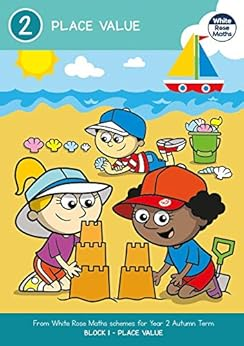## Year 2 PLACE VALUE Autumn Term BLOCK 1 - White Rose Maths

From White Rose Maths schemes for Year 2 Autumn Term BLOCK 1 – PLACE VALUE. The activities are designed to meet the aims of the national curriculum for mathematics: - become fluent in the fundamentals of mathematics, including through varied and frequent practice with increasingly complex problems over time, so that pupils develop conceptual understanding and the ability to recall and apply knowledge rapidly and accurately. - reason mathematically by following a line of enquiry, conjecturing relationships and generalisations, and developing an argument, justification or proof using mathematical language - can solve problems by applying their mathematics to a variety of routine and non-routine problems with increasing sophistication, including breaking down problems into a series of simpler steps and persevering in seeking solutions. Curriculum link for Year 2: - count in steps of 2, 3, and 5 from 0, and in tens from any number, forward andBackward - recognise the place value of each digit in a two-digit number (tens, ones) - identify, represent and estimate numbers using different representations, including the number line - compare and order numbers from 0 up to 100; use <, > and = signs - read and write numbers to at least 100 in numerals and in worduse place value and number facts to solve problems.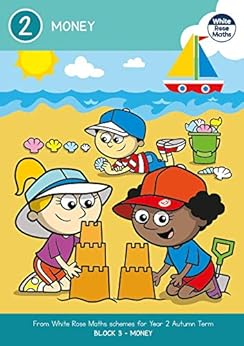## Year 2 MONEY Autumn Term BLOCK 3 - White Rose Maths

From White Rose Maths schemes for Year 2 Autumn Term BLOCK 3 – MONEY. The activities are designed to meet the aims of the national curriculum for mathematics: - become fluent in the fundamentals of mathematics, including through varied and frequent practice with increasingly complex problems over time, so that pupils develop conceptual understanding and the ability to recall and apply knowledge rapidly and accurately. - reason mathematically by following a line of enquiry, conjecturing relationships and generalisations, and developing an argument, justification or proof using mathematical language - can solve problems by applying their mathematics to a variety of routine and non-routine problems with increasing sophistication, including breaking down problems into a series of simpler steps and persevering in seeking solutions. Curriculum link for Year 2: - choose and use appropriate standard units to estimate and measure length/height in any direction (m/cm); mass (kg/g); temperature (°C); capacity (litres/ml) to the nearest appropriate unit, using rulers, scales, thermometers and measuring vessels - compare and order lengths, mass, volume/capacity and record the results using >,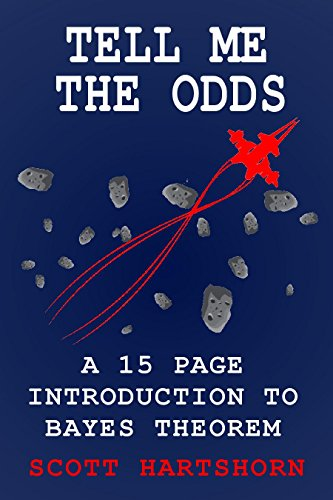## Tell Me The Odds: A 15 Page Introduction To Bayes Theorem

Bayes Theorem Is ImportantBayes Theorem is a way of updating probability as you get new information. Essentially, you make an initial guess, and then get more data to improve it. Bayes Theorem, or Bayes Rule, has a ton of real world applications, from estimating your risk of a heart attack to making recommendations on NetflixBut It Isn't That ComplicatedThis book is a short introduction to Bayes Theorem. It is only 15 pages long, and is intended to show you how Bayes Theorem works as quickly as possible. The examples are intentionally kept simple to focus solely on Bayes Theorem without requiring that the reader know complicated probability distributions.If you want to learn the basics of Bayes Theorem as quickly as possible, with some easy to duplicate examples, this is a good book for you.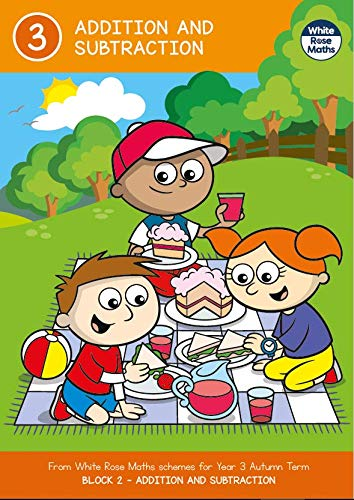## Year 3 ADDITION AND SUBTRACTION Autumn Term BLOCK 2 - White Rose Maths

From White Rose Maths schemes for Year 3 Autumn Term BLOCK 2 - ADDITION AND SUBTRACTION. The activities are designed to meet the aims of the national curriculum for mathematics: - become fluent in the fundamentals of mathematics, including through varied and frequent practice with increasingly complex problems over time, so that pupils develop conceptual understanding and the ability to recall and apply knowledge rapidly and accurately. - reason mathematically by following a line of enquiry, conjecturing relationships and generalisations, and developing an argument, justification or proof using mathematical language - can solve problems by applying their mathematics to a variety of routine and non-routine problems with increasing sophistication, including breaking down problems into a series of simpler steps and persevering in seeking solutions. Curriculum link for Year 3: - add and subtract numbers mentally, including: ○ a three-digit number and ones ○ a three-digit number and tens ○ a three-digit number and hundreds - add and subtract numbers with up to three digits, using formal written methods of columnar addition and subtraction - estimate the answer to a calculation and use inverse operations to check answerssolve problems, including missing number problems, using number facts, place value, and more complex addition and subtraction.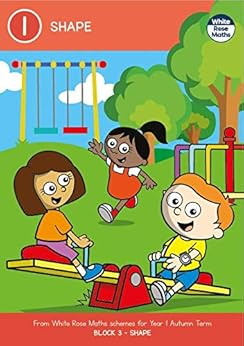## Year 1 SHAPE Autumn Term BLOCK 3 - White Rose Maths

From White Rose Maths schemes for Year 1 Autumn Term BLOCK 3 – SHAPE. The activities are designed to meet the aims of the national curriculum for mathematics: - become fluent in the fundamentals of mathematics, including through varied and frequent practice with increasingly complex problems over time, so that pupils develop conceptual understanding and the ability to recall and apply knowledge rapidly and accurately. - reason mathematically by following a line of enquiry, conjecturing relationships and generalisations, and developing an argument, justification or proof using mathematical language - can solve problems by applying their mathematics to a variety of routine and non-routine problems with increasing sophistication, including breaking down problems into a series of simpler steps and persevering in seeking solutions. Curriculum link for Year 1: - recognise and name common 2-D and 3-D shapes, including: ○ 2-D shapes [for example, rectangles (including squares), circles and triangles]3-D shapes [for example, cuboids (including cubes), pyramids and spheres].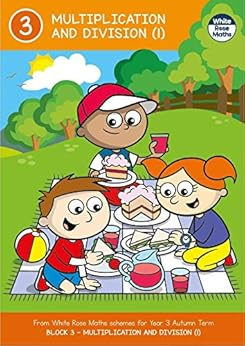## Year 3 MULTIPLICATION AND DIVISION (1) Autumn Term BLOCK 3 - White Rose Maths

From White Rose Maths schemes for Year 3 Autumn Term BLOCK 3 - MULTIPLICATION AND DIVISION (1). The activities are designed to meet the aims of the national curriculum for mathematics: - become fluent in the fundamentals of mathematics, including through varied and frequent practice with increasingly complex problems over time, so that pupils develop conceptual understanding and the ability to recall and apply knowledge rapidly and accurately. - reason mathematically by following a line of enquiry, conjecturing relationships and generalisations, and developing an argument, justification or proof using mathematical language - can solve problems by applying their mathematics to a variety of routine and non-routine problems with increasing sophistication, including breaking down problems into a series of simpler steps and persevering in seeking solutions. Curriculum link for Year 3: - recall and use multiplication and division facts for the 3, 4 and 8 multiplication tables - write and calculate mathematical statements for multiplication and division using the multiplication tables that they know, including for two-digit numbers times one-digit numbers, using mental and progressing to formal written methodssolve problems, including missing number problems, involving multiplication and division, including positive integer scaling problems and correspondence problems in which n objects are connected to m objects.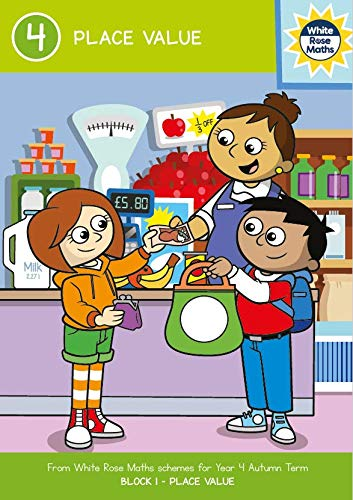## Year 4 PLACE VALUE Autumn Term BLOCK 1 a?? White Rose Maths

From White Rose Maths schemes for Year 4 Autumn Term BLOCK 1 – PLACE VALUE. The activities are designed to meet the aims of the national curriculum for mathematics: - become fluent in the fundamentals of mathematics, including through varied and frequent practice with increasingly complex problems over time, so that pupils develop conceptual understanding and the ability to recall and apply knowledge rapidly and accurately. - reason mathematically by following a line of enquiry, conjecturing relationships and generalisations, and developing an argument, justification or proof using mathematical language - can solve problems by applying their mathematics to a variety of routine and non-routine problems with increasing sophistication, including breaking down problems into a series of simpler steps and persevering in seeking solutions. Curriculum link for Year 4: - count in multiples of 6, 7, 9, 25 and 1000 - find 1000 more or less than a given number - count backwards through zero to include negative numbers - recognise the place value of each digit in a four-digit number (thousands, hundreds, tens, and ones) - order and compare numbers beyond 1000 - identify, represent and estimate numbers using different representations - round any number to the nearest 10, 100 or 1000 - solve number and practical problems that involve all of the above and with increasingly large positive numbersread Roman numerals to 100 (I to C) and know that over time, the numeral system changed to include the concept of zero and place value.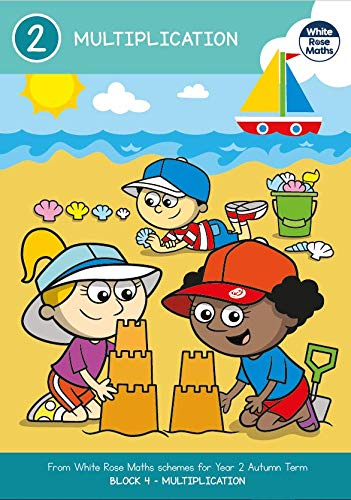## Year 2 MULTIPLICATION Autumn Term BLOCK 4 - White Rose Maths

From White Rose Maths schemes for Year 2 Autumn Term BLOCK 4 – MULTIPLICATION. The activities are designed to meet the aims of the national curriculum for mathematics: - become fluent in the fundamentals of mathematics, including through varied and frequent practice with increasingly complex problems over time, so that pupils develop conceptual understanding and the ability to recall and apply knowledge rapidly and accurately. - reason mathematically by following a line of enquiry, conjecturing relationships and generalisations, and developing an argument, justification or proof using mathematical language - can solve problems by applying their mathematics to a variety of routine and non-routine problems with increasing sophistication, including breaking down problems into a series of simpler steps and persevering in seeking solutions. Curriculum link for Year 2: - recall and use multiplication and division facts for the 2, 5 and 10 multiplication tables, including recognising odd and even numbers - calculate mathematical statements for multiplication and division within the multiplication tables and write them using the multiplication (×), division (÷) and equals (=) signs - show that multiplication of two numbers can be done in any order (commutative) and division of one number by another cannotsolve problems involving multiplication and division, using materials, arrays, repeated addition, mental methods, and multiplication and division facts, including problems in contexts.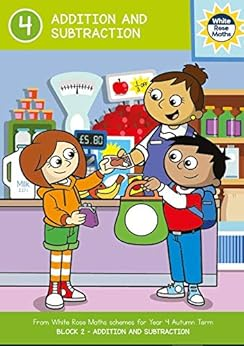## Year 4 ADDITION AND SUBTRACTION Autumn Term BLOCK 2 a?? White Rose Maths

From White Rose Maths schemes for Year 4 Autumn Term BLOCK 2 – ADDITION AND SUBTRACTION. The activities are designed to meet the aims of the national curriculum for mathematics: - become fluent in the fundamentals of mathematics, including through varied and frequent practice with increasingly complex problems over time, so that pupils develop conceptual understanding and the ability to recall and apply knowledge rapidly and accurately. - reason mathematically by following a line of enquiry, conjecturing relationships and generalisations, and developing an argument, justification or proof using mathematical language - can solve problems by applying their mathematics to a variety of routine and non-routine problems with increasing sophistication, including breaking down problems into a series of simpler steps and persevering in seeking solutions. Curriculum link for Year 4: - add and subtract numbers with up to 4 digits using the formal written methods of columnar addition and subtraction where appropriate - estimate and use inverse operations to check answers to a calculationsolve addition and subtraction two-step problems in contexts, deciding which operations and methods to use and why.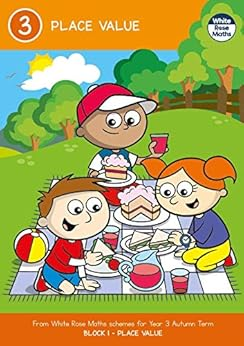## Year 3 PLACE VALUE Autumn Term BLOCK 1 - White Rose Maths

From White Rose Maths schemes for Year 3 Autumn Term BLOCK 1 – PLACE VALUE. The activities are designed to meet the aims of the national curriculum for mathematics: - become fluent in the fundamentals of mathematics, including through varied and frequent practice with increasingly complex problems over time, so that pupils develop conceptual understanding and the ability to recall and apply knowledge rapidly and accurately. - reason mathematically by following a line of enquiry, conjecturing relationships and generalisations, and developing an argument, justification or proof using mathematical language - can solve problems by applying their mathematics to a variety of routine and non-routine problems with increasing sophistication, including breaking down problems into a series of simpler steps and persevering in seeking solutions. Curriculum link for Year 3: - count from 0 in multiples of 4, 8, 50 and 100; find 10 or 100 more or less than a given number - recognise the place value of each digit in a three-digit number (hundreds, tens, ones) - compare and order numbers up to 1000 - identify, represent and estimate numbers using different representations - read and write numbers up to 1000 in numerals and in wordssolve number problems and practical problems involving these ideas.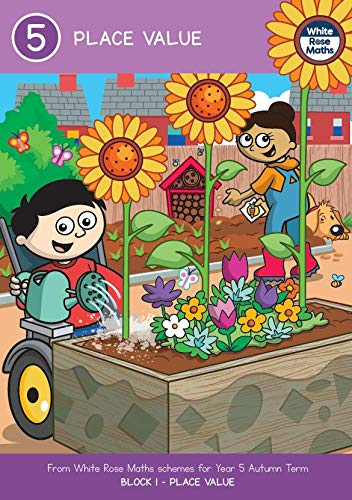## Year 5 PLACE VALUE Autumn Term BLOCK 1 a?? White Rose Maths

From White Rose Maths schemes for Year 5 Autumn Term BLOCK 1 – PLACE VALUE. The activities are designed to meet the aims of the national curriculum for mathematics: - become fluent in the fundamentals of mathematics, including through varied and frequent practice with increasingly complex problems over time, so that pupils develop conceptual understanding and the ability to recall and apply knowledge rapidly and accurately. - reason mathematically by following a line of enquiry, conjecturing relationships and generalisations, and developing an argument, justification or proof using mathematical language - can solve problems by applying their mathematics to a variety of routine and non-routine problems with increasing sophistication, including breaking down problems into a series of simpler steps and persevering in seeking solutions. Curriculum link for Year 5: - read, write, order and compare numbers to at least 1 000 000 and determine the value of each digit - count forwards or backwards in steps of powers of 10 for any given number up to 1 000 000 - interpret negative numbers in context, count forwards and backwards with positive and negative whole numbers, including through zero - round any number up to 1 000 000 to the nearest 10, 100, 1000, 10 000 and 100 000 - solve number problems and practical problems that involve all of the aboveread Roman numerals to 1000 (M) and recognise years written in Roman numerals.